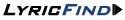The Kills - Ticket Man lyrics | LyricsFreak

# Ticket Man Lyrics

## The Kills – Ticket Man Lyrics

from album: No Wow (2005)
Here's the ticket, what's the problem
Too many tickets is the problem, man
Here's the ticket, what's the problem
Too many problems is the ticket in my hand
Why don't you give it up, get it down
Spend half as much, kills you in half the time
Oh pull on the baby, red number make it hard to hear
Hard to see and hard to sit around
Nothing's gonna kiss you like the kiss that's gonna get to you
From waiting it out

Here's the ticket, what's the problem
Too many tickets is the problem, man
Here's the ticket, what's the problem
Too many problems is the ticket, man
Here's the ticket, what's the problem
Too many tickets is the problem, man
Here's the ticket, what's the problem
Too many problems is the ticket in my hand

Why don't you give it up, get it down
Spend half as much, kills you in half the time
Oh it's a little too easy to always be kind
But the longer it takes, the more you must find
Oh it's a little too easy to always be kind
But the longer it takes, the more you must find

Here's the ticket, what's the problem
Too many tickets is the problem, man
Here's the ticket, what's the problem
Too many problems is the ticket, man
Here's the ticket, what's the problem
Too many tickets is the problem, man
Here's the ticket, what's the problem
Too many problems is the ticket in my hand
Share lyrics
×
Songwriters: JAMIE HINCE, ALISON MOSSHART
Ticket Man lyrics © EMI Music Publishing, Sony/ATV Music Publishing LLCLyrics term of use